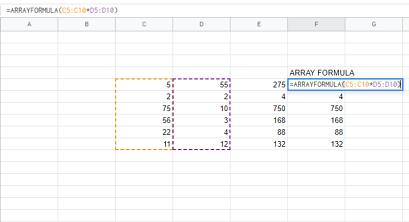# What Does \$ Mean in Google Sheets?

If you’ve been using Google Sheets for a while, you might have noticed the ‘\$’ symbol in the main menu. Moreover, why do you sometimes see the ‘\$’ symbol inserted in a formula?If you’ve been wondering why, it’s time to solve the confusion. In this guide, you’ll learn what ‘\$’ means in Google Sheets and how to use it. Keep reading to find out more.

## ‘\$’ Symbol in the Menu

Google Sheets is an easy solution for inserting various numerical data. You can use it to organize your phone numbers, important dates, and lists of ingredients. Furthermore, Google Sheets allows users to keep track of expenses, bills, or employees’ salaries.

However, to avoid confusion and know the numbers represent currencies, it’s important to write a currency sign. When you’re dealing with multiple numbers and cells, it can be bothersome to press the symbol on the keyboard every time you need it. This is why the ‘\$’ symbol in the menu is useful.

Clicking on this button enables the currency option. Therefore, the next number you write will be a currency. If you have multiple numbers across the cells, it’s easy to turn them into currencies by using this option.

## Number Formatting

When you head over to ‘Custom number format’ you may notice the dollar symbol. Clicking on any of the options means that that currencies in the spreadsheet will assume that number formatting. Remember that they don’t even need to have a ‘\$’ symbol before you tap on this option.

## ‘\$’ Symbols in Formula

Occasionally when you use Google Sheets, you want some variables to change and some to stay the same. But do you have to manually edit the formula every time you need to do this? Luckily, no. By using the absolute reference, users decide what parts of the formula they want to keep.

Moreover, this solution enables users to have control over the formulas they write. Let’s say you want to multiply the data from two different cells. You’ll type something like this ‘B1*C1.’ If you want to copy the result from one cell into another, you’ll get a new formula.

But sometimes you want some variables to stay the same, which is why you insert the ‘\$’ symbol. There are three different methods you can follow. Take a look at them:

### Placing the ‘\$’ Symbol on the Right in the Formula

If you want the data from the row to stay fixed, then placing the ‘\$’ symbol on the right will do the trick. For example, if you want to copy the formula from one cell and paste it to others, insert ‘\$’ before the row number.

Doing so ensures that when you copy the formula to any other cell on the spreadsheet, the reference will always be the original row. However, the column will change depending on where you paste the formula.

### Placing the ‘\$’ Symbol on the Left in the Formula

If you need the column to stay fixed, then insert the ‘\$’ symbol on the left. More precisely, write the symbol immediately after the equation sign. With this formula, the column will be locked, but the rows will update relative to the cell location.

### Double ‘\$’ Symbol in the Formula

Do you want both the row and the column to remain constant? Then, insert the double dollar symbol in the formula. For instance, writing ‘\$A1\$’ ensures the rows and columns remain the same, no matter where you paste the formula.

Besides the Absolute Reference formula, there are many other formulas that can make your life easier. In this section, we’ll explore some of the most commonly used formulas every Google Sheets user should know.### Changing References to Absolute Using the Shortcut

Do you know there’s a faster way to change the reference from relative to absolute without typing the dollar symbol? How do you do this? Simple, if you use Windows, press the ‘F4’ key. Mac users should simultaneously press ‘F4’ and ‘Fn.’ This effective shortcut allows you to toggle between the relative and absolute reference effortlessly.

### Array Formula

An Array formula is one of the most commonly used formulas. It’s useful when you’re dealing with a larger database. The beauty of it is that any time you add new information, it’ll be included automatically.

Let’s say you want to multiply the values of two cells. Take a look at this example:To apply the formula, you’ll type ‘=C5*D5.’ You can drag it down the cell and get the correct results for each cell you multiplied.

By inserting ‘ARRAYFORMULA,’ at the beginning, you will ensure that any updates to the spreadsheet are visible in the cell where you applied this formula. Let’s first apply this formula. Write the ‘ARRAYFORMULA’ in the formula bar, select the whole C row and multiply it by the whole D row. It’ll look like this:Now, let’s say you need to add a new row between C5 and C6 and D5 and D6. Once you write the values in these cells, there’s no need to apply a new multiplying formula. The Array formula does this for you.## Various ‘\$’ Functions

As you can see, the ‘\$’ symbol has various meanings. The most obvious is that it represents the currency. However, you can also use it in formulas to keep some parts of variables. Which formulas do you use the most? Share some tips in the comments section below.

Disclaimer: Some pages on this site may include an affiliate link. This does not effect our editorial in any way.

Todays Highlights